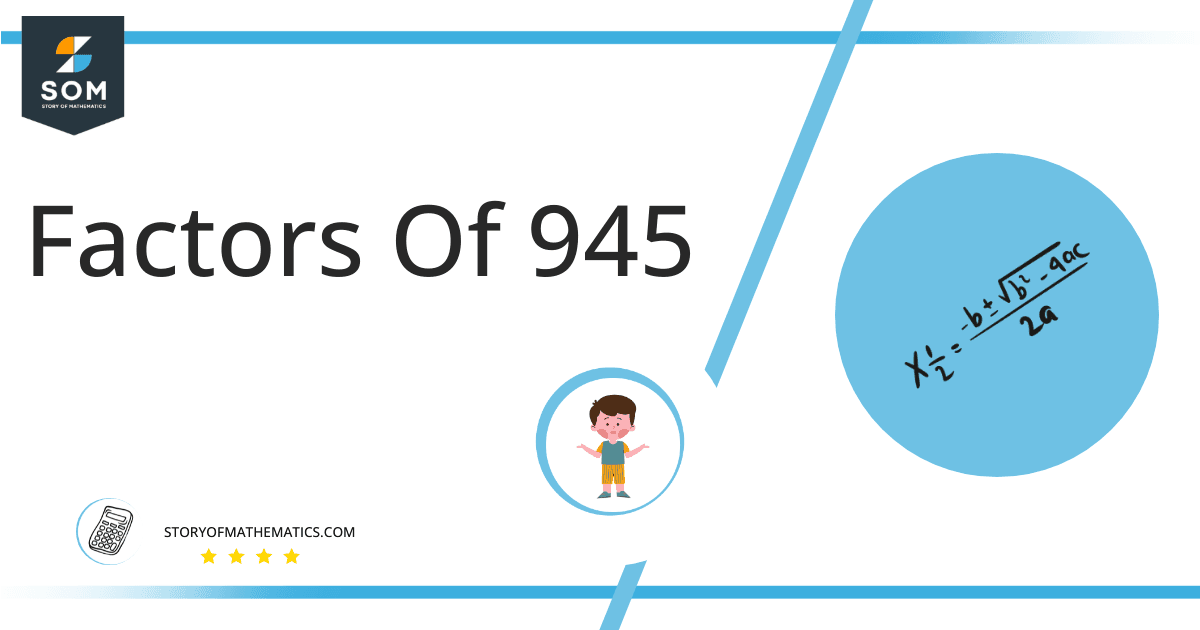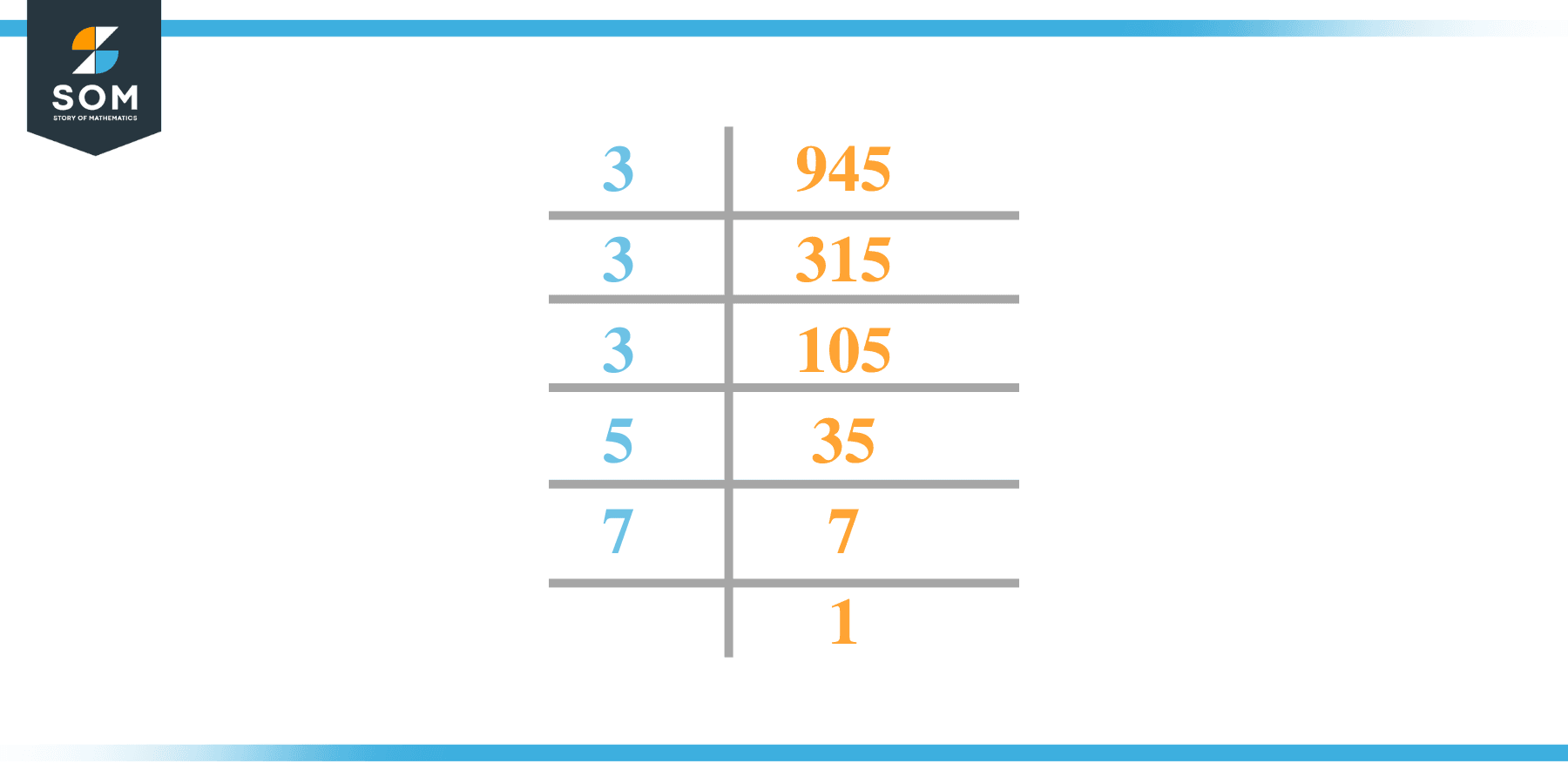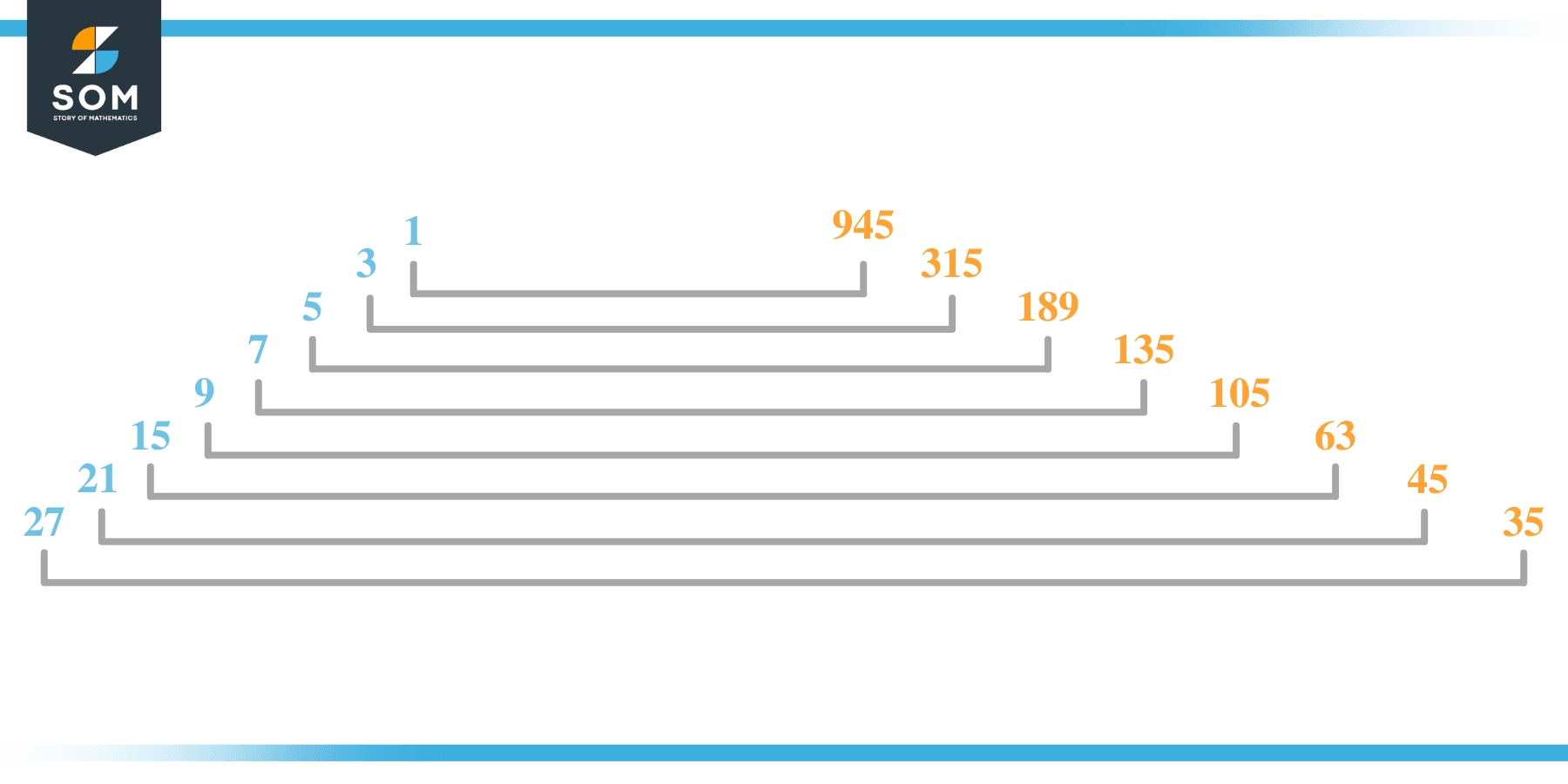# Factors of 945: Prime Factorization, Methods, and Examples

We’ll look at many 945-related issues in this article. First, we see that the factors are those numbers that result from multiplying the factors of a number. For example, in this case, 3, 5, and 7 are prime factors of 945.Hence the result of multiplying 3x3x3x5x7 is 945.

### Factors of 945

Here are the factors of number 945.

Factors of 945: 1, 3, 5, 7, 9, 15, 21, 27, 35, 45, 63, 105, 135, 189, 315 and 945

### Negative Factors of 945

The negative factors of 945 are similar to their positive aspects, just with a negative sign.

Negative Factors of 945: -1, -3, -5, -7, -9, -15, -21, -27, -35, -45, -63, -105, -135, -189, -315 and -945

### Prime Factorization of 945

The prime factorization of 945 is the way of expressing its prime factors in the product form.

Prime Factorization: 33 x 51 x 71

In this article, we will learn about the factors of 945 and how to find them using various techniques such as upside-down division, prime factorization, and factor tree.

## What Are the Factors of 945?

The factors of 945 are 1, 3, 5, 7, 9, 15, 21, 27, 35, 45, 63, 105, 135, 189, 315 and 945. These numbers are the factors as they do not leave any remainder when divided by 945.

The factors of 945 are classified as prime numbers and composite numbers. The prime factors of the number 945 can be determined using the prime factorization technique.

## How To Find the Factors of 945?

You can find the factors of 945 by using the rules of divisibility. The divisibility rule states that any number, when divided by any other natural number, is said to be divisible by the number if the quotient is the whole number and the resulting remainder is zero.

To find the factors of 945, create a list containing the numbers that are exactly divisible by 945 with zero remainders. One important thing to note is that 1 and 945 are the 945’s factors as every natural number has 1 and the number itself as its factor.

1 is also called the universal factor of every number. The factors of 945 are determined as follows:

$\dfrac{945}{1} = 945$

$\dfrac{945}{3} = 315$

$\dfrac{945}{5} = 189$

$\dfrac{945}{7} = 135$

$\dfrac{945}{9} = 105$

$\dfrac{945}{15} = 63$

$\dfrac{945}{21} = 45$

$\dfrac{945}{27} = 35$

$\dfrac{945}{35} = 27$

$\dfrac{945}{45} = 21$

$\dfrac{945}{63} = 15$

$\dfrac{945}{105} = 9$

$\dfrac{945}{135} =7$

$\dfrac{945}{189} = 5$

$\dfrac{945}{315} = 3$

$\dfrac{945}{945} = 1$

Therefore,1, 3, 5, 7, 9, 15, 21, 27, 35, 45, 63, 105, 135, 189, 315 and 945 are the factors of 945.

### Total Number of Factors of 945

For 945, there are 16 positive factors and 16 negative ones. So in total, there are 32 factors out of 945.

To find the total number of factors of the given number, follow the procedure mentioned below:

1. Find the factorization/prime factorization of the given number.
2. Demonstrate the prime factorization of the number in the form of exponent form.
3. Add 1 to each of the exponents of the prime factor.
4. Now, multiply the resulting exponents together. This obtained product is equivalent to the total number of factors of the given number.

By following this procedure, the total number of factors of 945 is given as:

Factorization of 945 is 33 x 51 x 71.

The exponent of 3 is 3, 5 is 1, and 7 is 1.

Adding 1 to each and multiplying them together results in 32.

Therefore, the total number of factors of 945 is 32. 16 are positive, and 16 factors are negative.

### Important Notes

Here are some essential points that must be considered while finding the factors of any given number:

• The factor of any given number must be a whole number.
• The factors of the number cannot be in the form of decimals or fractions.
• Factors can be positive as well as negative.
• Negative factors are the additive inverse of the positive factors of a given number.
• The factor of a number cannot be greater than that number.
• Every even number has 2 as its prime factor, the smallest prime factor.

## Factors of 945 by Prime FactorizationThe number 945 is a composite number. Prime factorization is a valuable technique for finding the number’s prime factors and expressing the number as the product of its prime factors.

Before finding the factors of 945 using prime factorization, let us find out what prime factors are. Prime factors are the factors of any given number that are only divisible by 1 and themselves.

To start the prime factorization of 945, start dividing by its most minor prime factor. First, determine that the given number is either even or odd. If it is an even number, then 2 will be the smallest prime factor.

Continue splitting the quotient obtained until 1 is received as the quotient. The prime factorization of 945 can be expressed as:

945 = 33 x 51 x 71

## Factors of 945 in PairsThe factor pairs are the duplet of numbers that, when multiplied together, result in the factorized number. Factor pairs can be more than one depending on the total number of factors given.

For 945, the factor pairs can be found as:

1 x 945 = 945

3 x 315 = 945

5 x189 = 945

7 x 135 = 945

9 x 105 = 945

15 x 63 = 945

21 x 45 = 945

27 x 35 = 945

The possible factor pairs of 945 are given as (1, 945)(3, 315)(5, 189)(7, 135)(9, 105)(15, 63)(21, 45) and(27, 35).

All these numbers in pairs, when multiplied, give 945 as the product.

The negative factor pairs of 945 are given as:

-1 x -945 = 945

-3 x -315 = 945

-5 x -189 = 945

-7 x -135 = 945

-9 x -105 = 945

-15 x -63 = 945

-21 x -45 = 945

-27 x -35 = 945

It is important to note that in negative factor pairs, the minus sign has been multiplied by the minus sign, due to which the resulting product is the original positive number. Therefore, -1, -3, -5, -7, -9, -15, -21, -27, -35, -45, -63, -105, -135, -189, -315 and -945 are called negative factors of 945.

The list of all the factors of 945, including positive as well as negative numbers, is given below.

Factor list of 945: 1,-1, 3,-3, 5,-5, 7,-7, 9,-9, 15,-15, 21,-21, 27,-27, 35,-35, 45,-45, 63,-63, 105,-105, 135,-135, 189,-189, 315,-315 , 945, and -945

## Factors of 945 Solved Examples

To better understand the concept of factors, let’s solve some examples.

### Example 1

How many factors of 945 are there?

### Solution

The total number of Factors of 945 is 32.

Factors of 945 are 1, 3, 5, 7, 9, 15, 21, 27, 35, 45, 63, 105, 135, 189, 315 and 945.

### Example 2

Find the factors of 945 using prime factorization.

### Solution

The prime factorization of 945 is given as:

945 $\div$ 3 = 315

315 $\div$ 3 = 105

105 $\div$ 3 = 35

35  $\div$ 5 = 7

7 $\div$ 7 = 1

So the prime factorization of 945 can be written as:

33 x 51 x 71 = 945